# Resources tagged with: Multiplication & division

Filter by: Content type:
Age range:
Challenge level:

### There are 153 results

Broad Topics > Calculations and Numerical Methods > Multiplication & division### What Two ...?

##### Age 7 to 11 ShortChallenge Level

56 406 is the product of two consecutive numbers. What are these two numbers?### Tom's Number

##### Age 7 to 11Challenge Level

Work out Tom's number from the answers he gives his friend. He will only answer 'yes' or 'no'.### Divide it Out

##### Age 7 to 11Challenge Level

What is the lowest number which always leaves a remainder of 1 when divided by each of the numbers from 2 to 10?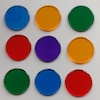### Dicey Array

##### Age 5 to 11Challenge Level

Watch the video of this game being played. Can you work out the rules? Which dice totals are good to get, and why?### Ducking and Dividing

##### Age 7 to 11Challenge Level

Your vessel, the Starship Diophantus, has become damaged in deep space. Can you use your knowledge of times tables and some lightning reflexes to survive?### Product Sudoku

##### Age 11 to 16Challenge Level

The clues for this Sudoku are the product of the numbers in adjacent squares.### Oh! Hidden Inside?

##### Age 11 to 14Challenge Level

Find the number which has 8 divisors, such that the product of the divisors is 331776.### A First Product Sudoku

##### Age 7 to 14Challenge Level

Given the products of adjacent cells, can you complete this Sudoku?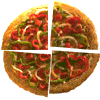### Fair Feast

##### Age 5 to 11Challenge Level

Here is a picnic that Petros and Michael are going to share equally. Can you tell us what each of them will have?### Thirty Six Exactly

##### Age 11 to 14Challenge Level

The number 12 = 2^2 × 3 has 6 factors. What is the smallest natural number with exactly 36 factors?### Being Resilient - Primary Number

##### Age 5 to 11Challenge Level

Number problems at primary level that may require resilience.### Code Breaker

##### Age 7 to 11Challenge Level

This problem is based on a code using two different prime numbers less than 10. You'll need to multiply them together and shift the alphabet forwards by the result. Can you decipher the code?### Eminit

##### Age 11 to 14Challenge Level

The number 8888...88M9999...99 is divisible by 7 and it starts with the digit 8 repeated 50 times and ends with the digit 9 repeated 50 times. What is the value of the digit M?### The Remainders Game

##### Age 7 to 14Challenge Level

Play this game and see if you can figure out the computer's chosen number.### Multiples Sudoku

##### Age 11 to 14Challenge Level

Each clue in this Sudoku is the product of the two numbers in adjacent cells.### Factoring Factorials

##### Age 11 to 14Challenge Level

Find the highest power of 11 that will divide into 1000! exactly.### Times Right

##### Age 11 to 16Challenge Level

Using the digits 1, 2, 3, 4, 5, 6, 7 and 8, mulitply a two two digit numbers are multiplied to give a four digit number, so that the expression is correct. How many different solutions can you find?### Book Codes

##### Age 7 to 11Challenge Level

Look on the back of any modern book and you will find an ISBN code. Take this code and calculate this sum in the way shown. Can you see what the answers always have in common?### Powerful Factorial

##### Age 11 to 14Challenge Level

6! = 6 x 5 x 4 x 3 x 2 x 1. The highest power of 2 that divides exactly into 6! is 4 since (6!) / (2^4 ) = 45. What is the highest power of two that divides exactly into 100!?### Missing Multipliers

##### Age 7 to 14Challenge Level

What is the smallest number of answers you need to reveal in order to work out the missing headers?### Journeys on the Gattegno Tens Chart

##### Age 5 to 11

Alf describes how the Gattegno chart helped a class of 7-9 year olds gain an awareness of place value and of the inverse relationship between multiplication and division.### Jumping

##### Age 7 to 11Challenge Level

After training hard, these two children have improved their results. Can you work out the length or height of their first jumps?### A Conversation Piece

##### Age 7 to 11Challenge Level

Take the number 6 469 693 230 and divide it by the first ten prime numbers and you'll find the most beautiful, most magic of all numbers. What is it?### Funny Factorisation

##### Age 11 to 16Challenge Level

Using the digits 1 to 9, the number 4396 can be written as the product of two numbers. Can you find the factors?### Remainders

##### Age 7 to 14Challenge Level

I'm thinking of a number. My number is both a multiple of 5 and a multiple of 6. What could my number be?### What Is Ziffle?

##### Age 7 to 11Challenge Level

Can you work out what a ziffle is on the planet Zargon?### Making Pathways

##### Age 7 to 11Challenge Level

Can you find different ways of creating paths using these paving slabs?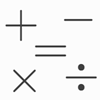### Which Symbol?

##### Age 7 to 11Challenge Level

Choose a symbol to put into the number sentence.### Magic Potting Sheds

##### Age 11 to 14Challenge Level

Mr McGregor has a magic potting shed. Overnight, the number of plants in it doubles. He'd like to put the same number of plants in each of three gardens, planting one garden each day. Can he do it?### Gabriel's Problem

##### Age 11 to 14Challenge Level

Gabriel multiplied together some numbers and then erased them. Can you figure out where each number was?### Repeaters

##### Age 11 to 14Challenge Level

Choose any 3 digits and make a 6 digit number by repeating the 3 digits in the same order (e.g. 594594). Explain why whatever digits you choose the number will always be divisible by 7, 11 and 13.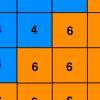### Six Ten Total

##### Age 7 to 11Challenge Level

This challenge combines addition, multiplication, perseverance and even proof.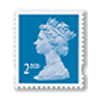### Penta Post

##### Age 7 to 11Challenge Level

Here are the prices for 1st and 2nd class mail within the UK. You have an unlimited number of each of these stamps. Which stamps would you need to post a parcel weighing 825g?### Difficulties with Division

##### Age 5 to 11

This article for teachers looks at how teachers can use problems from the NRICH site to help them teach division.### Napier's Bones

##### Age 7 to 11Challenge Level

The Scot, John Napier, invented these strips about 400 years ago to help calculate multiplication and division. Can you work out how to use Napier's bones to find the answer to these multiplications?### Compare the Calculations

##### Age 7 to 11Challenge Level

Can you put these four calculations into order of difficulty? How did you decide?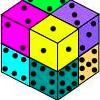### Six Numbered Cubes

##### Age 7 to 11Challenge Level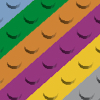### Looking at Lego

##### Age 7 to 11Challenge Level

This task offers an opportunity to explore all sorts of number relationships, but particularly multiplication.### Interactive Balance

##### Age 5 to 11Challenge Level

In this simulation of a balance, you can drag numbers and parts of number sentences on to the trays. Have a play!### The Pied Piper of Hamelin

##### Age 7 to 11Challenge Level

This problem is based on the story of the Pied Piper of Hamelin. Investigate the different numbers of people and rats there could have been if you know how many legs there are altogether!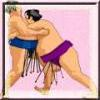### Sumo

##### Age 7 to 11Challenge Level

A number game requiring a strategy.### Dice and Spinner Numbers

##### Age 7 to 11Challenge Level

If you had any number of ordinary dice, what are the possible ways of making their totals 6? What would the product of the dice be each time?### X Is 5 Squares

##### Age 7 to 11Challenge Level

Can you arrange 5 different digits (from 0 - 9) in the cross in the way described?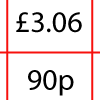### How Much Did it Cost?

##### Age 7 to 11Challenge Level

Use your logical-thinking skills to deduce how much Dan's crisps and ice-cream cost altogether.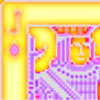### Wild Jack

##### Age 7 to 11Challenge Level

A game for 2 or more players with a pack of cards. Practise your skills of addition, subtraction, multiplication and division to hit the target score.### Number Tracks

##### Age 7 to 11Challenge Level

Benâ€™s class were cutting up number tracks. First they cut them into twos and added up the numbers on each piece. What patterns could they see?### Being Resourceful - Primary Number

##### Age 5 to 11Challenge Level

Number problems at primary level that require careful consideration.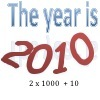### It Was 2010!

##### Age 5 to 11Challenge Level

If the answer's 2010, what could the question be?### More Playing with Numbers Upper Primary

##### Age 7 to 11

More resources to support understanding multiplication and division through playing with numbers### Going Round in Circles

##### Age 11 to 14Challenge Level

Mathematicians are always looking for efficient methods for solving problems. How efficient can you be?